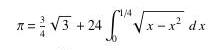﻿

## Homework 7 (due 3/11) CSC 431

This week we  finished chapter 6 (6.5.3), covered parts of chapter 7 (7.1, 7.3.1, 7.3.2, parts of 7.3.4/7.3.5) and chapter 8 (8.1, 8.3). Next week we will cover some of the remaining topics in chapter 8 and go on to chapter 9. Also, next week I will hand out the take-home final (due one week later).

During class we saw some of Heath's simulations:

and we discussed some of my solutions to homework 6: Bernoulli and the accuracy problem.

Submission: you can submit the homework by hardcopy in class or by sending it to me as an email.

Note: for all of the problems on this homework you can use the computer, but still explain  your steps.

1. [Horner's Scheme, 5pt] Rewrite f(x) 5x^4 - 10x^3 + 5x^2 - x + 7 using Horner's scheme and evaluate f(3) using Horner's scheme.

2. [Interpolation, 20pt] You have observed the following data:

 t y = f(t) -1 10 0 4 1 6 2 2
1. Using polynomial interpolation (Vandermonde matrix), find an interpolant in the monomoial basis.
2. Using Lagrange interpolation, find an interpolant in the Lagrange basis.
3. How many additions/multiplications are needed to evaluate each of the two interpolants (assuming that you pre-compute divisors in the Lagrange interpolant and evaluate the polynomial interpolant using Horner's scheme)?

3. [Integration, 25pt] Newton derived the following formula to compute pi:1. Use Midpoint, trapezoid and Simpson's quadrature rules to approximate the integral and find an approximation to pi. Compute errors and compare.
2. Using Gaussian quadrature with two and three points to approximate the integral and obtain an approximation to pi. We derived the locations of two points for the interval [-1,1]. See Gaussian quadrature for the locations of three points and how to change the interval from [-1,1] to [0,1/4]. Again, compute errors and compare (to the methods in a as well).
3. Review composite quadrature rules (Example 8.6 of the book) and approximate the integral using composite midpoint and trapezoid rules by breaking the interval into n subintervals. Try various values of n. Again compute errors; at what value of n, roughly, are the composite rules comparable to Gaussian quadrature with three points?
4. (Extra Credit) Derive the composite Simpson's rule for arbitrary n and apply it to approximate pi using the above formula. How does its accuracy compare to the composite midpoint and trapezoid rules?

Marcus Schaefer
Last updated: March 5th, 2010.